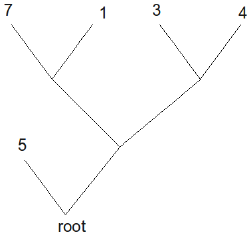## Problem S5: Nutrient Tree

There is a binary tree with l leaves (1 ≤ l ≤ 50) where each leaf k has an amount of nutrients nk (1 ≤ nk ≤ 10000) that it produces.

The branches (which you can think of as edges) of this binary tree limit the maximum amount of nutrients that can flow to the root of the tree. You have X growth agents (1 ≤ X ≤ 2500) that can be used to increase the thickness of an edge or increase the nutrient production of a leaf node. Initially, each edge has a weight of 1 and if you give it w growth agents then it can transport (1 + w)2 nutrients. Increasing the nutrient production of a leaf with initial value nk by s raises the nutrient production of that leaf to nk + s.

Notice that when edges meet, the amount of nutrient that flows is the sum of nutrients flowing along the incoming edges.

Find the maximum amount of nutrients you can transport to the root.

### Input

The first line of input will be a description of the tree. This description can be defined recursively as either an integer nk (1 ≤ nk ≤ 10000) or as (TLTR) where TL and TR are descriptions of the left and right subtrees, respectively. The second line of input will be the integer X, the amount of growth agents you have. Note: at least 30% of the marks for this question have l ≤ 5 and X ≤ 50.

### Output

On one line, output the maximum amount of nutrients that can flow into the root of the tree.

### Sample Input

```(5 ((7 1) (3 4)))
3```

`7`

### Explanation

The tree description can be illustrated as:Notice that if we put two growth factors on the left edge of the root, and one growth factor on the right edge of the root. Then, there will be 5 nutrients flowing from the leaf labelled 5 to the root (since the capacity is (1+2)2 = 9 units of nutrients) and 2 nutrients flowing from the right subtree (since the capacity is (1+1)2 = 4, but there are only 2 units of nutrients due to limits of 1 on the edges attached to all other leaves).

Alternatively, notice that increasing the nutrient production of the left leaf of the root to 6, and increasing the capacity of the root to the left leaf by 2 (to give a capacity of (1+2)2 = 9 units) would cause 6 units of nutrients to flow into the root from the left tree, and the right subtree of the root would produce 1 unit of nutrient.

In both cases, the maximum amount of nutrients that can reach the root node is 7.

Point Value: 20 (partial)
Time Limit: 2.00s
Memory Limit: 16M

Languages Allowed:
C++03, PAS, C, HASK, ASM, RUBY, PYTH2, JAVA, PHP, SCM, CAML, PERL, C#, C++11, PYTH3

``( 5 ( ( 7 1 ) ( 3 4 ) ) )``